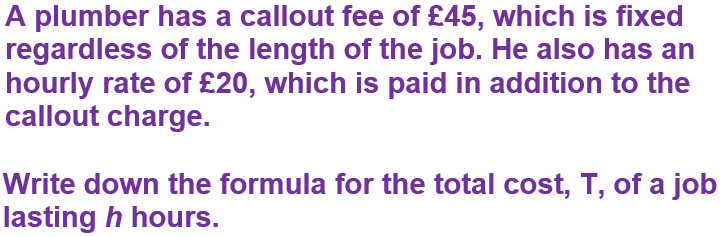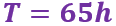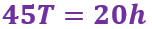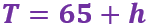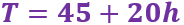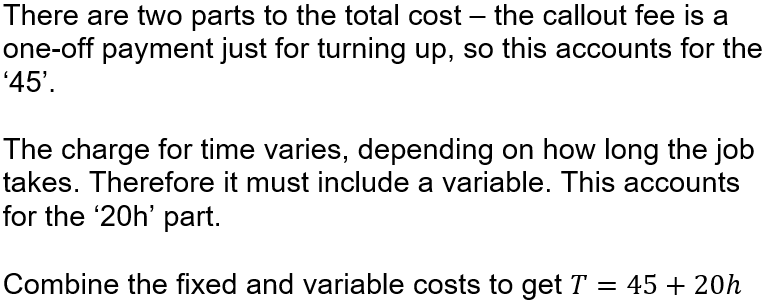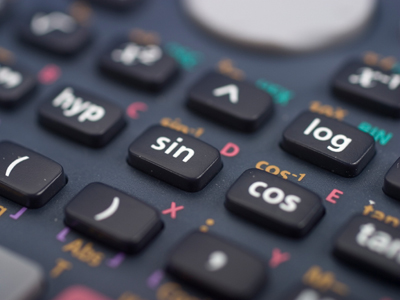See if you can get full marks in this maths quiz.

# Algebra 01 (F)

This GCSE Maths quiz will test your knowledge of the basic rules of Algebra.

Algebra is all about using symbols, usually letters, to represent a number that we don’t yet know. We call these letters VARIABLES, as their value can change, or vary, depending on the situation. A situation can be written down as an EXPRESSION – this can be thought of as a mathematical sentence. There are a few rules you need to know so that these expressions can be as neat and tidy as possible.

Imagine you had a number of squares and quarter-circles, and you can combine these to make different shapes. We can use the letter S to indicate a square, and Q for a quarter-circle.

Using 3 squares and 2 quarter-circles it is possible to make a number of different shapes, but each one will have the same area. We can write this as the expression 3S + 2Q.

Now imagine someone else comes along and gives you 2 squares and 4 quarter-circles. How many squares, and how many quarter-circles do you now have? It should be obvious that you have 5S + 6Q. You can’t add a square to a circle, and one of our rules of Algebra is that we can’t add terms that have different letters. The rules for multiplying and dividing are a bit different, so make sure you know the difference.

Having good Algebra skills opens up a whole world of opportunities, from Finance to Space Travel and everything in between!

Question 1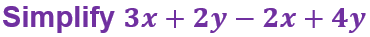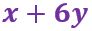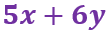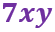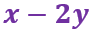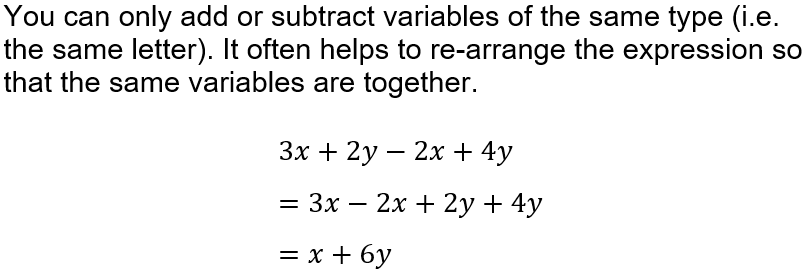Question 2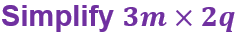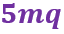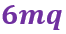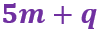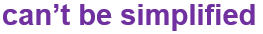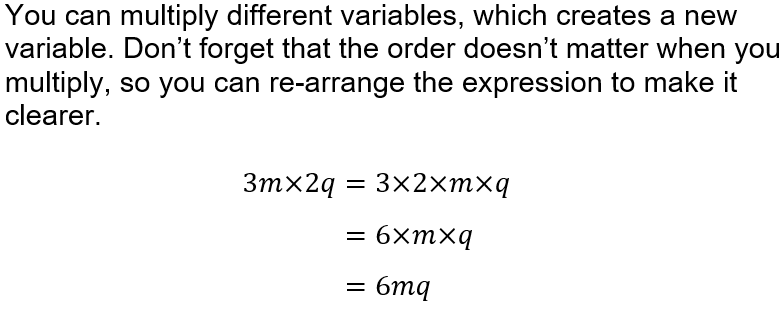Question 3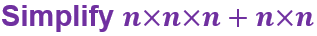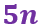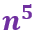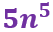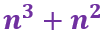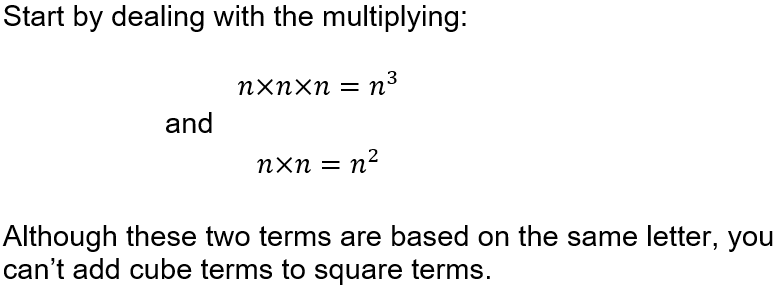Question 4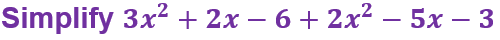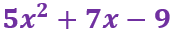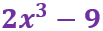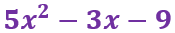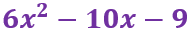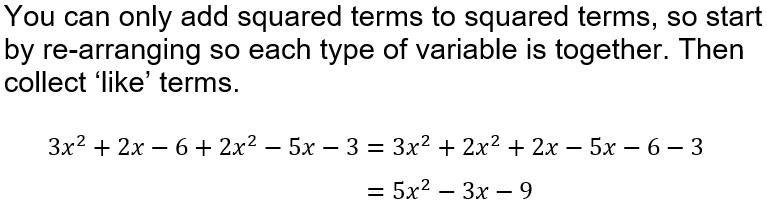Question 5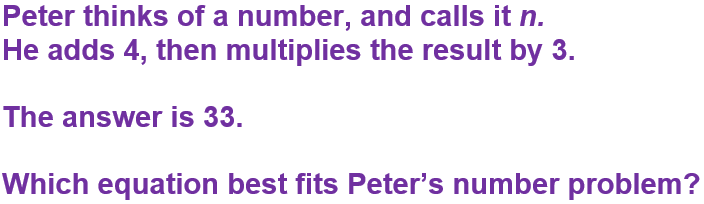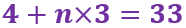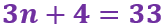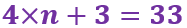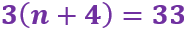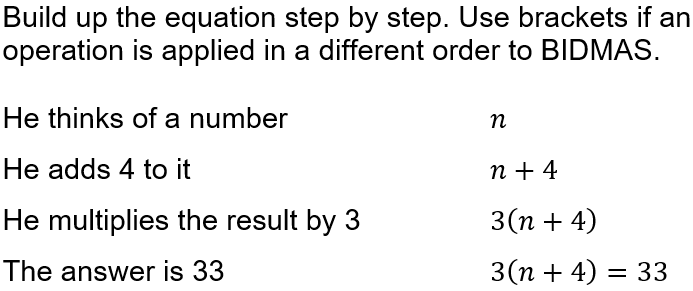Question 6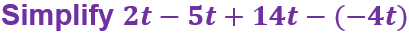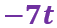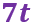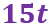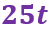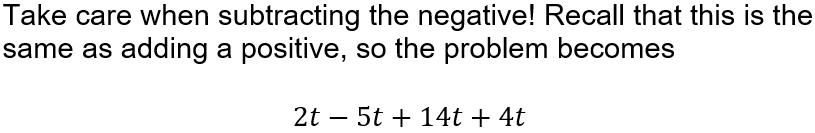Question 7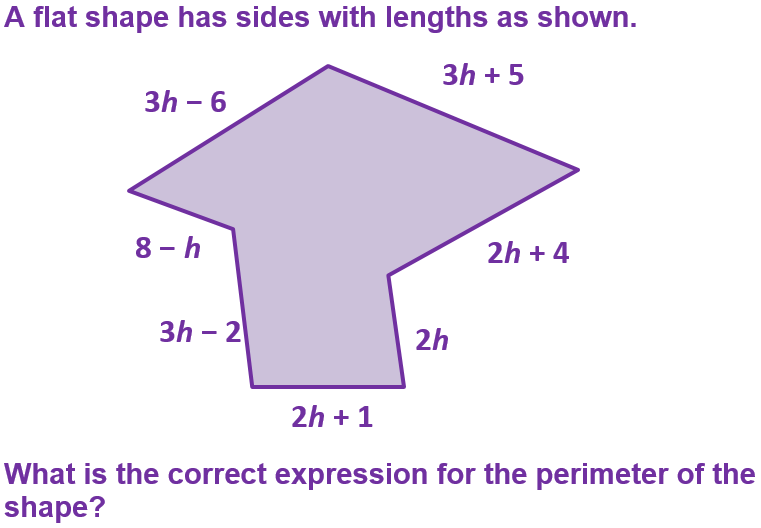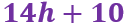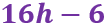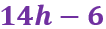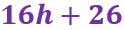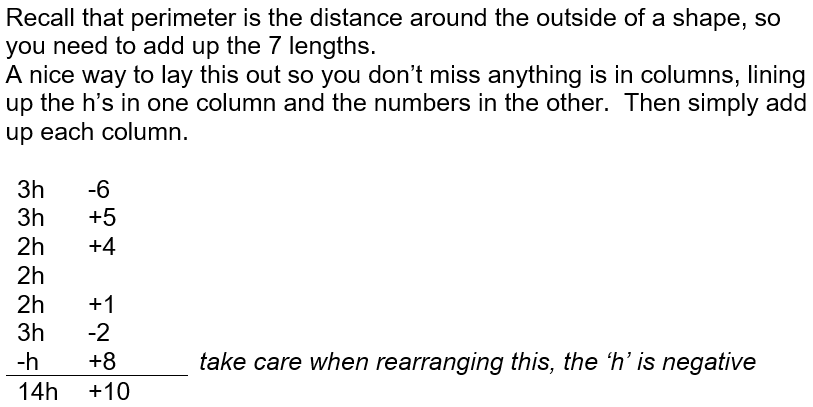Question 8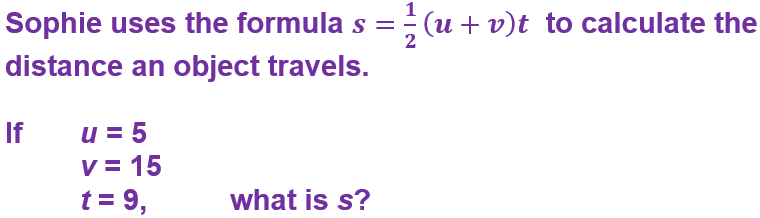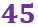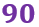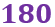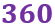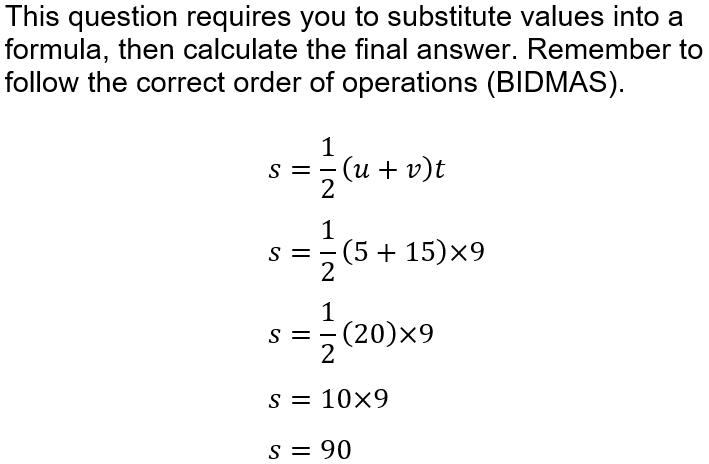Question 9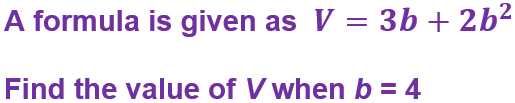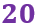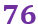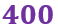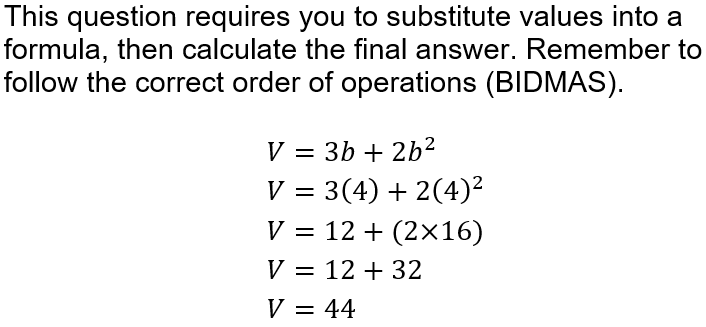Question 10